# 1 Reed’s problem

Reed’s problem as in

is a common test problem for transport codes. It is comprised of heterogeneous materials with strong absorber, vacuum, and scattering regions. These regions are valuable to testing different aspects of numerical discretizations.

PROBLEM neutron_sn DIM 1 GROUPS 1 SN $1 READ_MESH reed.msh MATERIAL source1 S1=50 Sigma_t1=50 Sigma_s1.1=0 MATERIAL absorber S1=0 Sigma_t1=5 Sigma_s1.1=0 MATERIAL void S1=0 Sigma_t1=0 Sigma_s1.1=0 MATERIAL source2 S1=1 Sigma_t1=1 Sigma_s1.1=0.9 MATERIAL reflector S1=0 Sigma_t1=1 Sigma_s1.1=0.9 BC left mirror BC right vacuum SOLVE_PROBLEM PRINT_FUNCTION phi1 $ gmsh -1 reed.geo
$[...]$ for n in 2 4 6 8; do feenox reed.fee ${n} | sort -g > reed-s${n}.csv; done
$ The solutions obtained in FeenoX with S_2, S_4, S_6 and S_8 are plotted and compared against and independent solution from https://www.drryanmc.com/solutions-to-reeds-problem/.Solution of the Reed’s problem # 2 Azmy’s problem As in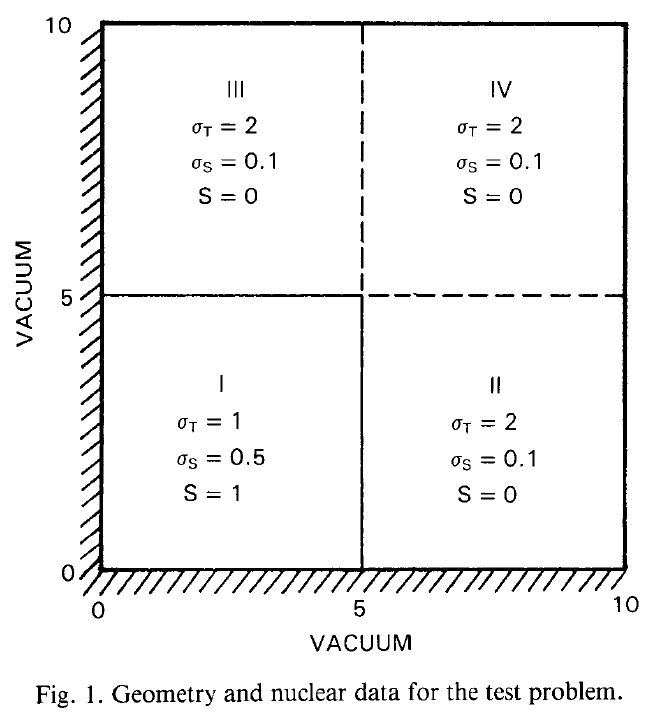Original problem formulation from 1988 paper ## 2.1 Second-order complete structured rectangular grid This example solves the problem using a structured second-order grid. It computes the mean flux in each quadrant by integrating \phi_1 over each physical group using the instruction INTEGRATE.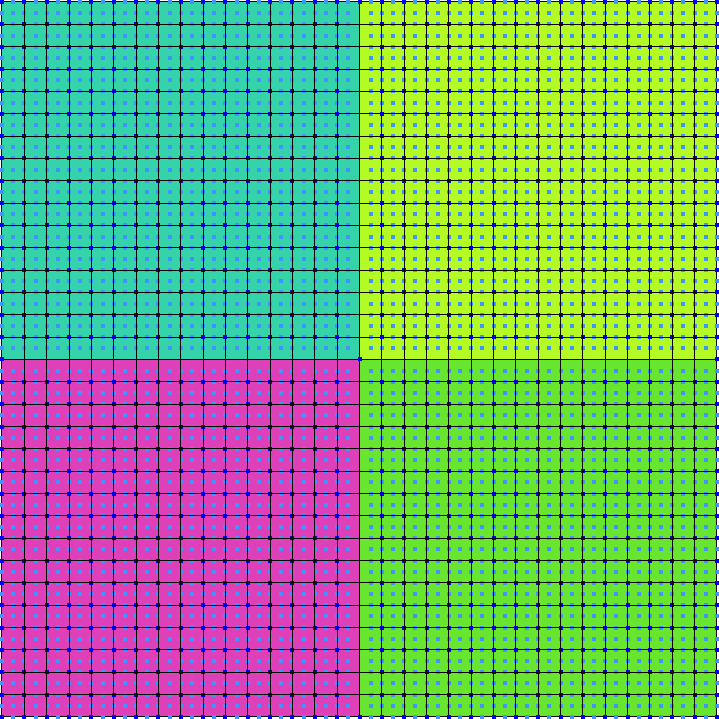Second-order complete structured rectangular grid DEFAULT_ARGUMENT_VALUE 1 4 PROBLEM neutron_sn DIM 2 GROUPS 1 SN$1

READ_MESH $0.msh MATERIAL src S1=1 Sigma_t1=1 Sigma_s1.1=0.5 MATERIAL abs S1=0 Sigma_t1=2 Sigma_s1.1=0.1 PHYSICAL_GROUP llq MATERIAL src PHYSICAL_GROUP lrq MATERIAL abs PHYSICAL_GROUP urq MATERIAL abs PHYSICAL_GROUP ulq MATERIAL abs BC mirror mirror BC vacuum vacuum SOLVE_PROBLEM # compute mean values in each quadrant INTEGRATE phi1 OVER llq RESULT lower_left_quadrant INTEGRATE phi1 OVER lrq RESULT lower_right_quadrant INTEGRATE phi1 OVER urq RESULT upper_right_quadrant PRINTF "LLQ = %.3e (ref 1.676e+0)\n" lower_left_quadrant/(5*5) PRINTF "LRQ = %.3e (ref 4.159e-2)\n" lower_right_quadrant/(5*5) PRINTF "URQ = %.3e (ref 1.992e-3)\n" upper_right_quadrant/(5*5) WRITE_RESULTS PRINTF "%g unknowns for S${1}, memory needed = %.1f Gb\n" total_dofs memory()
$gmsh -2 azmy-structured.geo$ feenox azmy-structured.fee 2
LLQ = 1.653e+00 (ref 1.676e+0)
LRQ = 4.427e-02 (ref 4.159e-2)
URQ = 2.712e-03 (ref 1.992e-3)
16900 unknowns for S2, memory needed = 0.2 Gb
$feenox azmy-structured.fee 4 LLQ = 1.676e+00 (ref 1.676e+0) LRQ = 4.164e-02 (ref 4.159e-2) URQ = 1.978e-03 (ref 1.992e-3) 50700 unknowns for S4, memory needed = 0.7 Gb$ feenox azmy-structured.fee 6
LLQ = 1.680e+00 (ref 1.676e+0)
LRQ = 4.120e-02 (ref 4.159e-2)
URQ = 1.874e-03 (ref 1.992e-3)
101400 unknowns for S6, memory needed = 2.7 Gb
$ ## 2.2 Fist-order locally-refined unstructured triangular grid This example solves the problem using an unstructured first-order grid. It computes the mean flux in each quadrant by integrating \phi_1 over x and y in custom ranges using the functional integral.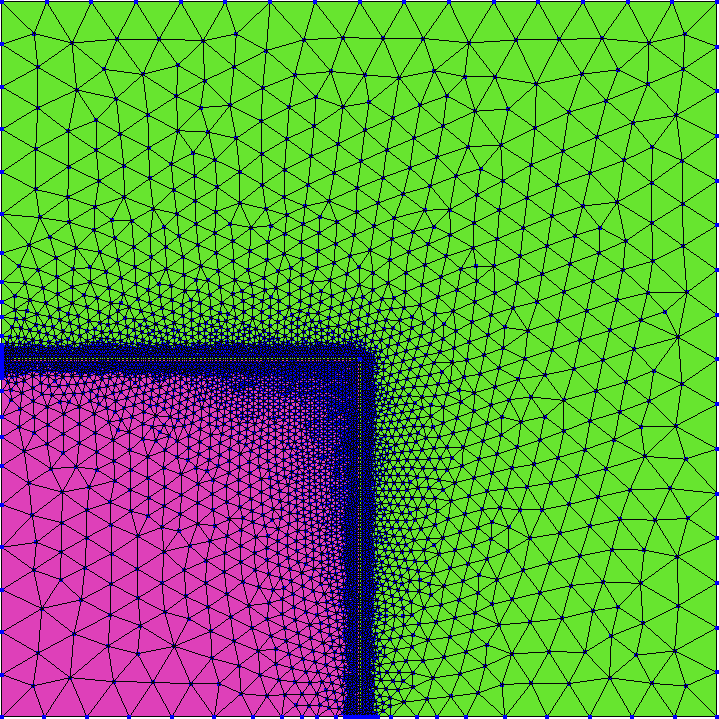Fist-order locally-refined unstructured triangular grid DEFAULT_ARGUMENT_VALUE 1 4 PROBLEM neutron_sn DIM 2 GROUPS 1 SN$1

READ_MESH $0.msh S1_src = 1 Sigma_t1_src = 1 Sigma_s1.1_src = 0.5 S1_abs = 0 Sigma_t1_abs = 2 Sigma_s1.1_abs = 0.1 BC mirror mirror BC vacuum vacuum # sn_alpha = 1 SOLVE_PROBLEM # compute mean values in each quadrant lower_left_quadrant = integral(integral(phi1(x,y),y,0,5),x,0,5)/(5*5) lower_right_quadrant = integral(integral(phi1(x,y),y,0,5),x,5,10)/(5*5) upper_right_quadrant = integral(integral(phi1(x,y),y,5,10),x,5,10)/(5*5) PRINT %.3e "LLQ" lower_left_quadrant "(ref 1.676e+0)" PRINT %.3e "LRQ" lower_right_quadrant "(ref 4.159e-2)" PRINT %.3e "URQ" upper_right_quadrant "(ref 1.992e-3)" # compute three profiles along x=constant profile5(y) = phi1(5.84375,y) profile7(y) = phi1(7.84375,y) profile9(y) = phi1(9.84375,y) PRINT_FUNCTION profile5 profile7 profile9 MIN 0 MAX 10 NSTEPS 100 FILE$0-$1.dat WRITE_RESULTS PRINTF "%g unknowns for S${1}, memory needed = %.1f Gb\n" total_dofs memory()
$gmsh -2 azmy.geo$ feenox azmy.fee 2
LLQ     1.653e+00       (ref 1.676e+0)
LRQ     4.427e-02       (ref 4.159e-2)
URQ     2.717e-03       (ref 1.992e-3)
15704 unknowns for S2, memory needed = 0.1 Gb
$feenox azmy.fee 4 LLQ 1.676e+00 (ref 1.676e+0) LRQ 4.160e-02 (ref 4.159e-2) URQ 1.991e-03 (ref 1.992e-3) 47112 unknowns for S4, memory needed = 0.5 Gb$ feenox azmy.fee 6
LLQ     1.680e+00       (ref 1.676e+0)
LRQ     4.115e-02       (ref 4.159e-2)
URQ     1.890e-03       (ref 1.992e-3)
94224 unknowns for S6, memory needed = 1.6 Gb
$feenox azmy.fee 8 LLQ 1.682e+00 (ref 1.676e+0) LRQ 4.093e-02 (ref 4.159e-2) URQ 1.844e-03 (ref 1.992e-3) 157040 unknowns for S8, memory needed = 4.3 Gb$ gmsh azmy-s4.geo
$gmsh azmy-s6.geo$ gmsh azmy-s8.geo
$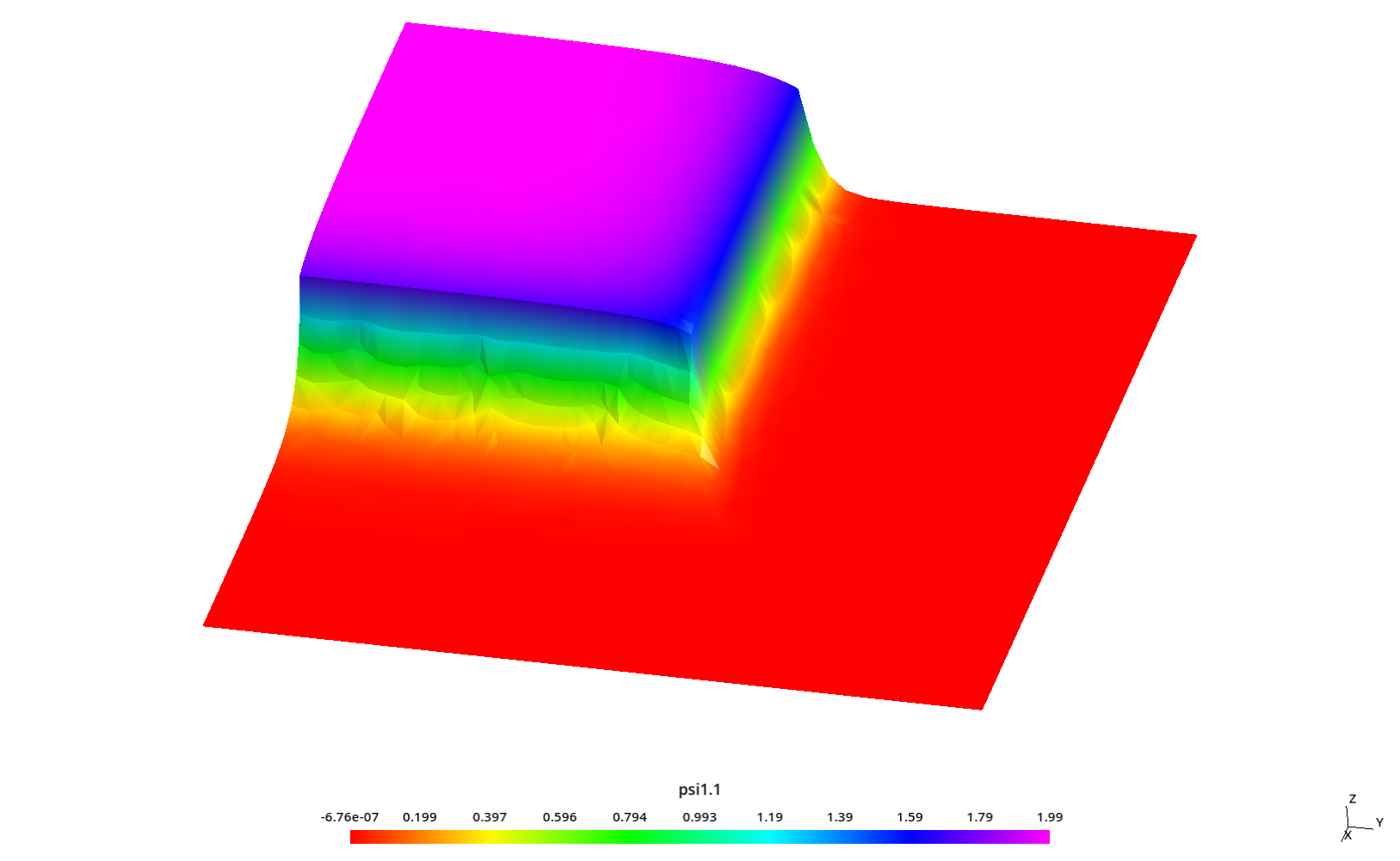\psi_{1,1} for S_4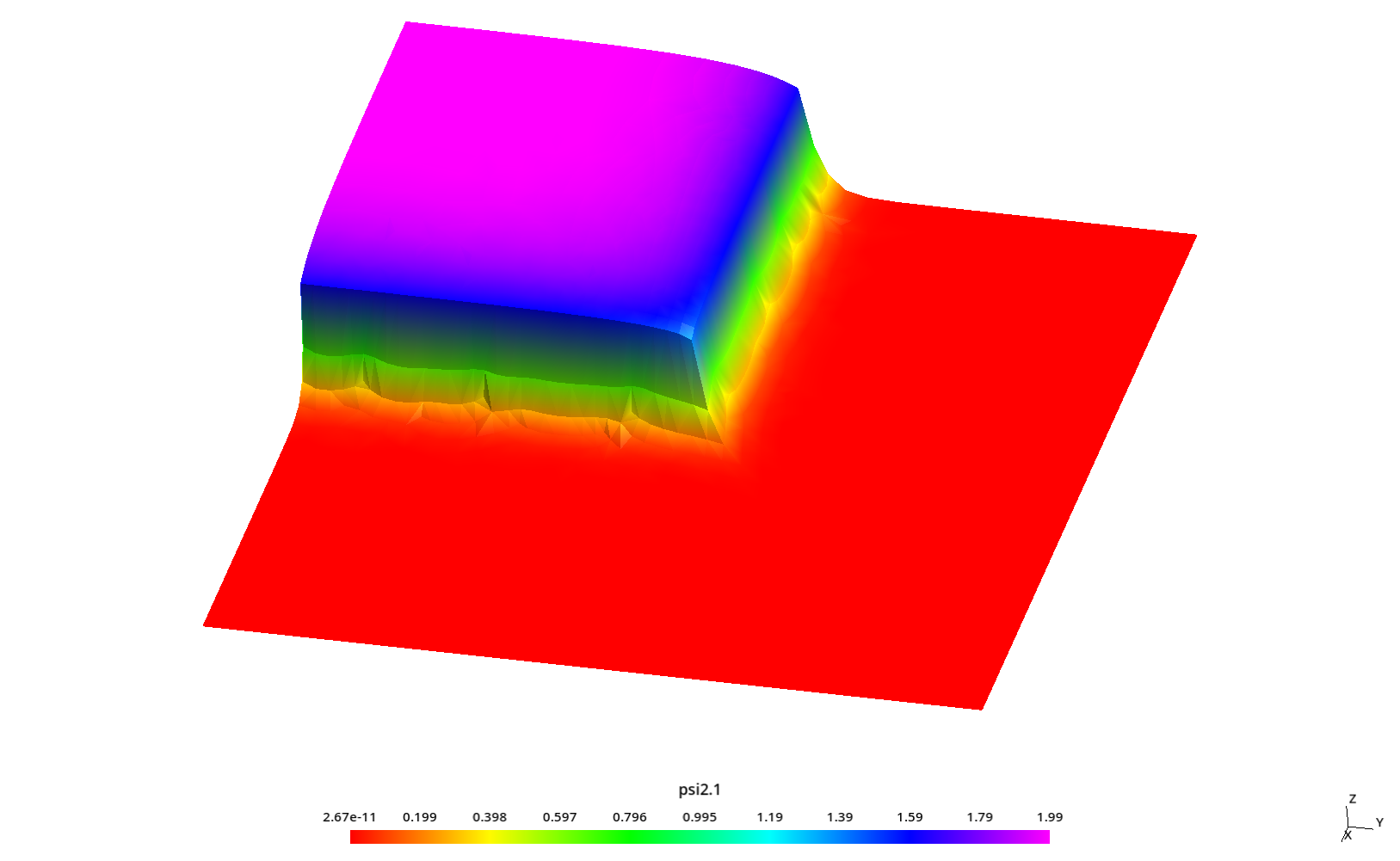\psi_{2,1} for S_4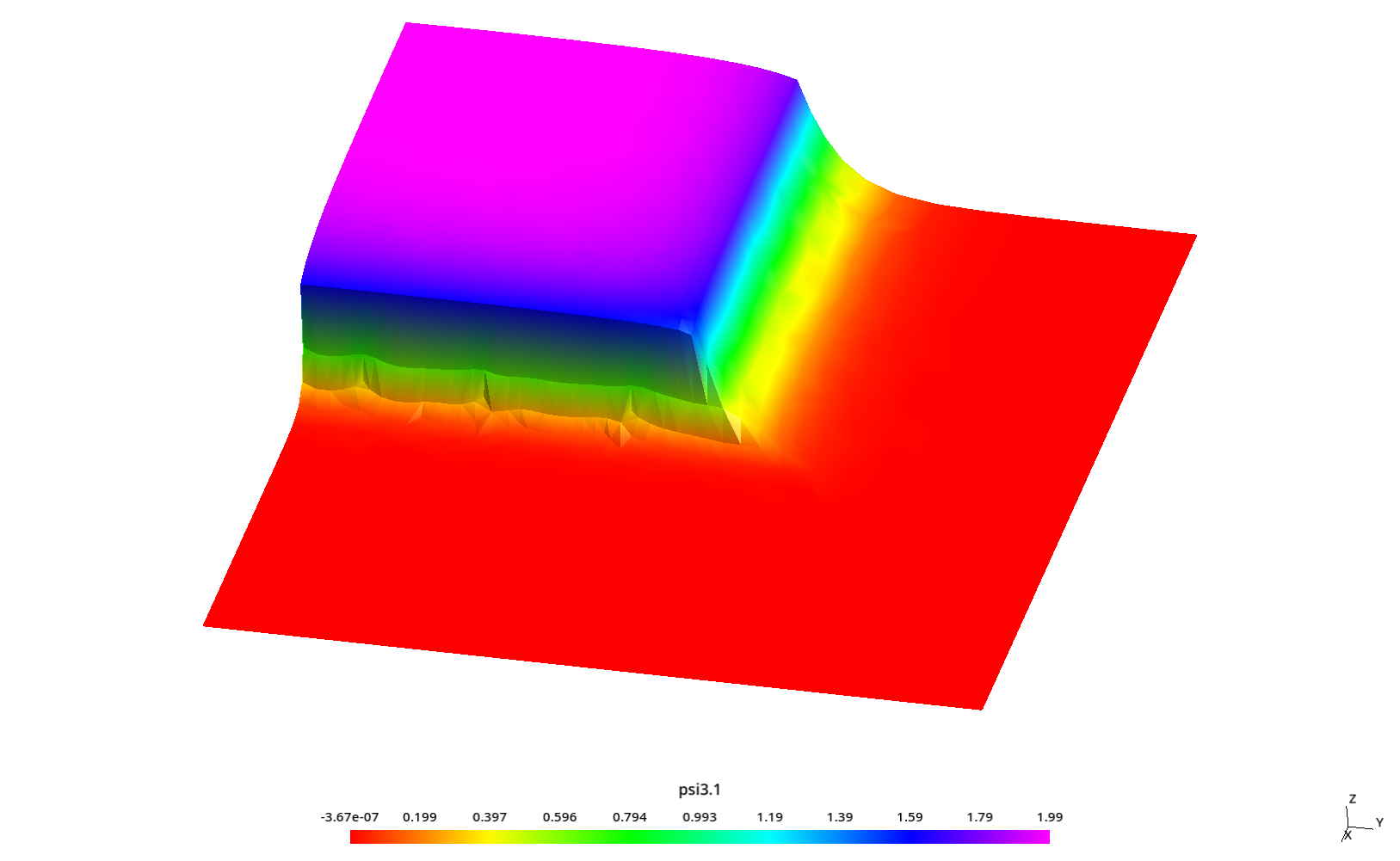\psi_{3,1} for S_4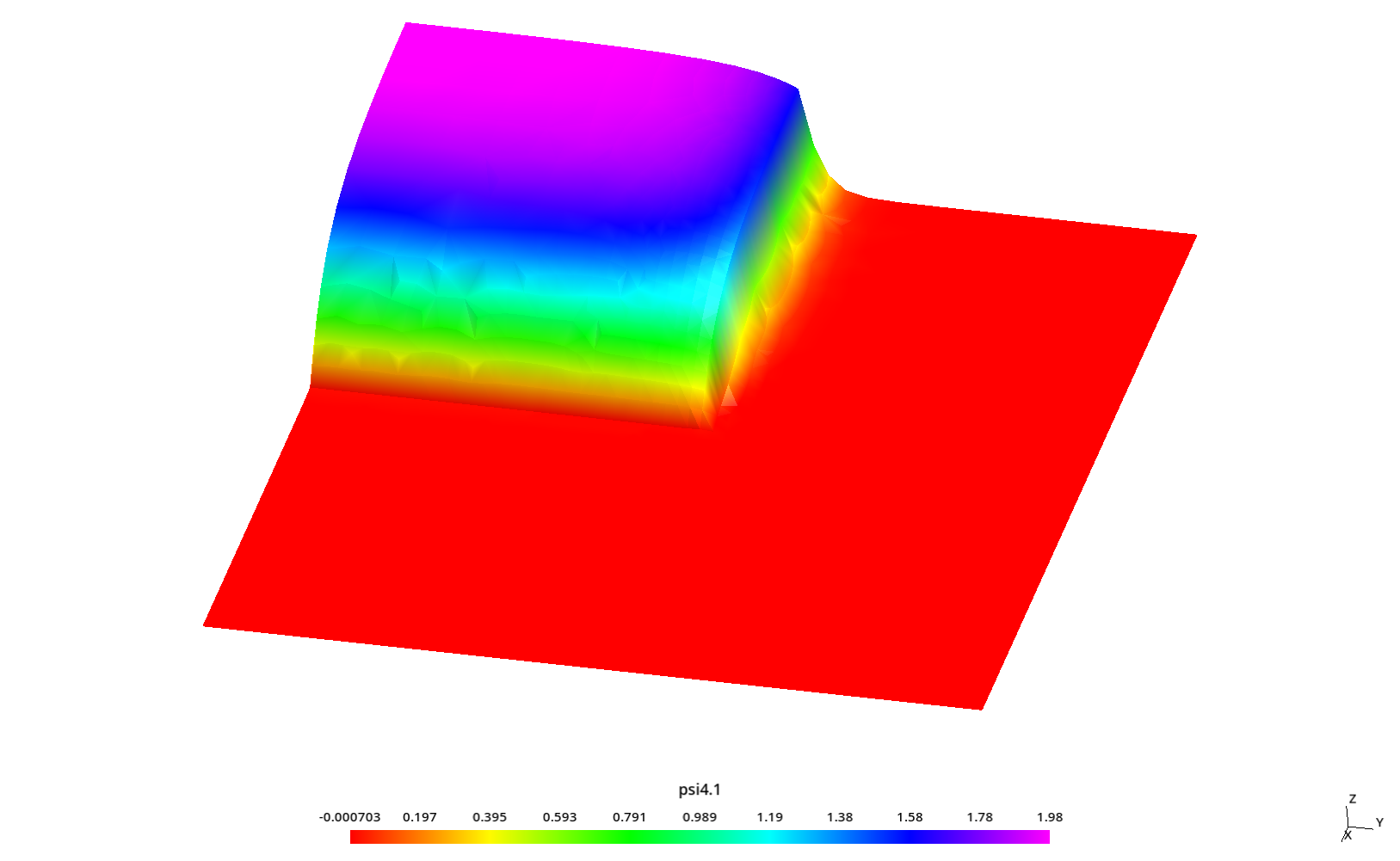\psi_{4,1} for S_4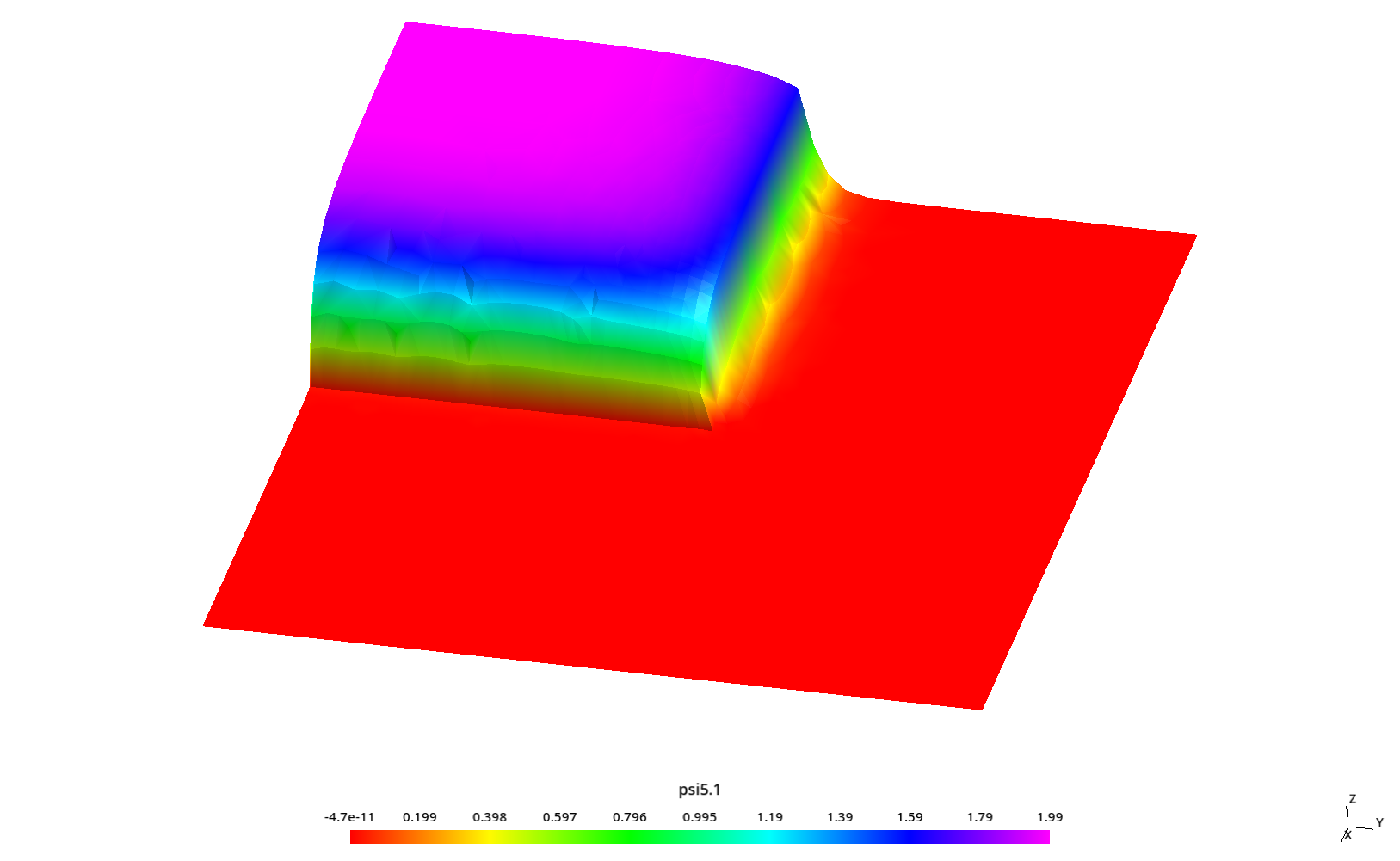\psi_{5,1} for S_4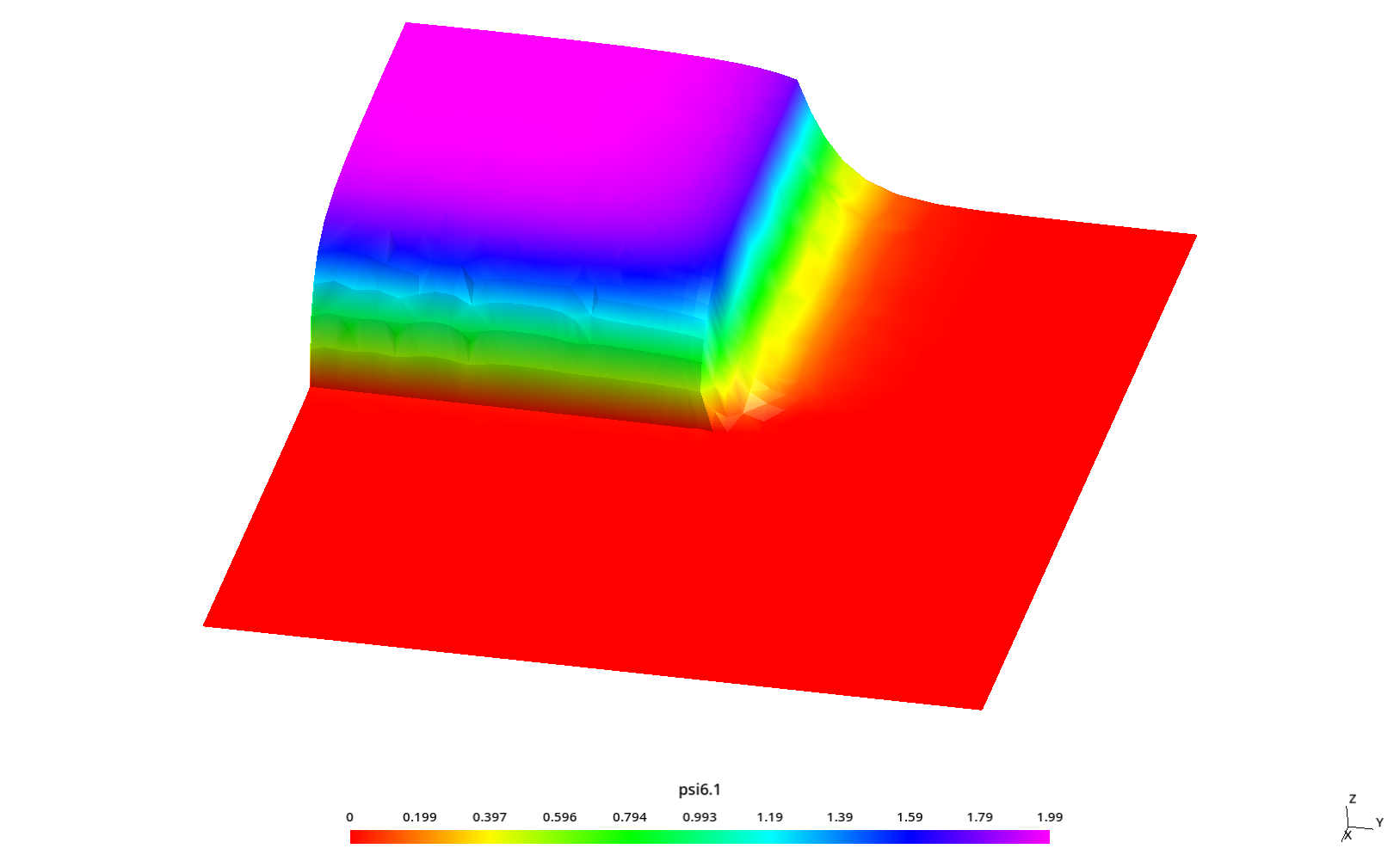\psi_{6,1} for S_4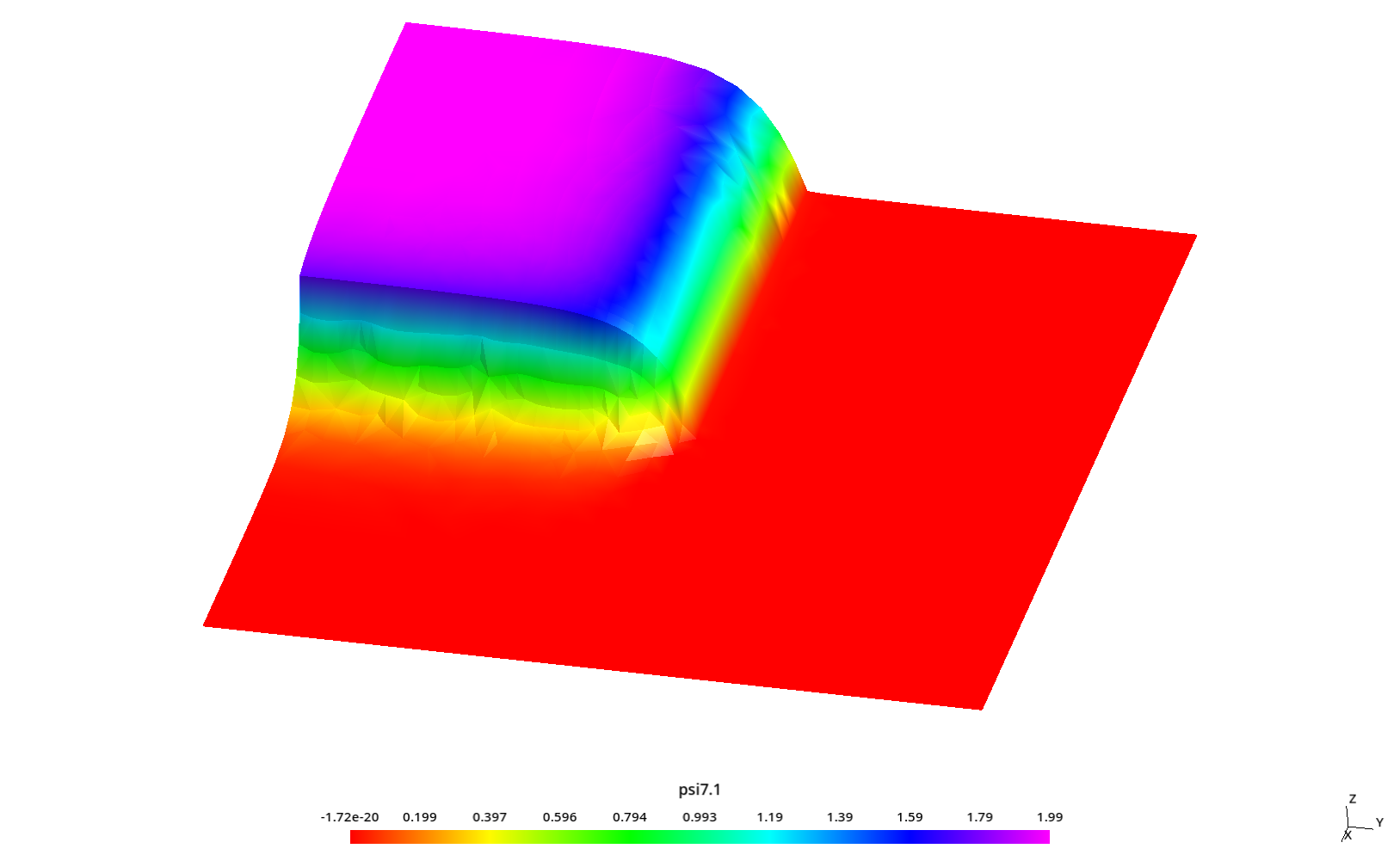\psi_{7,1} for S_4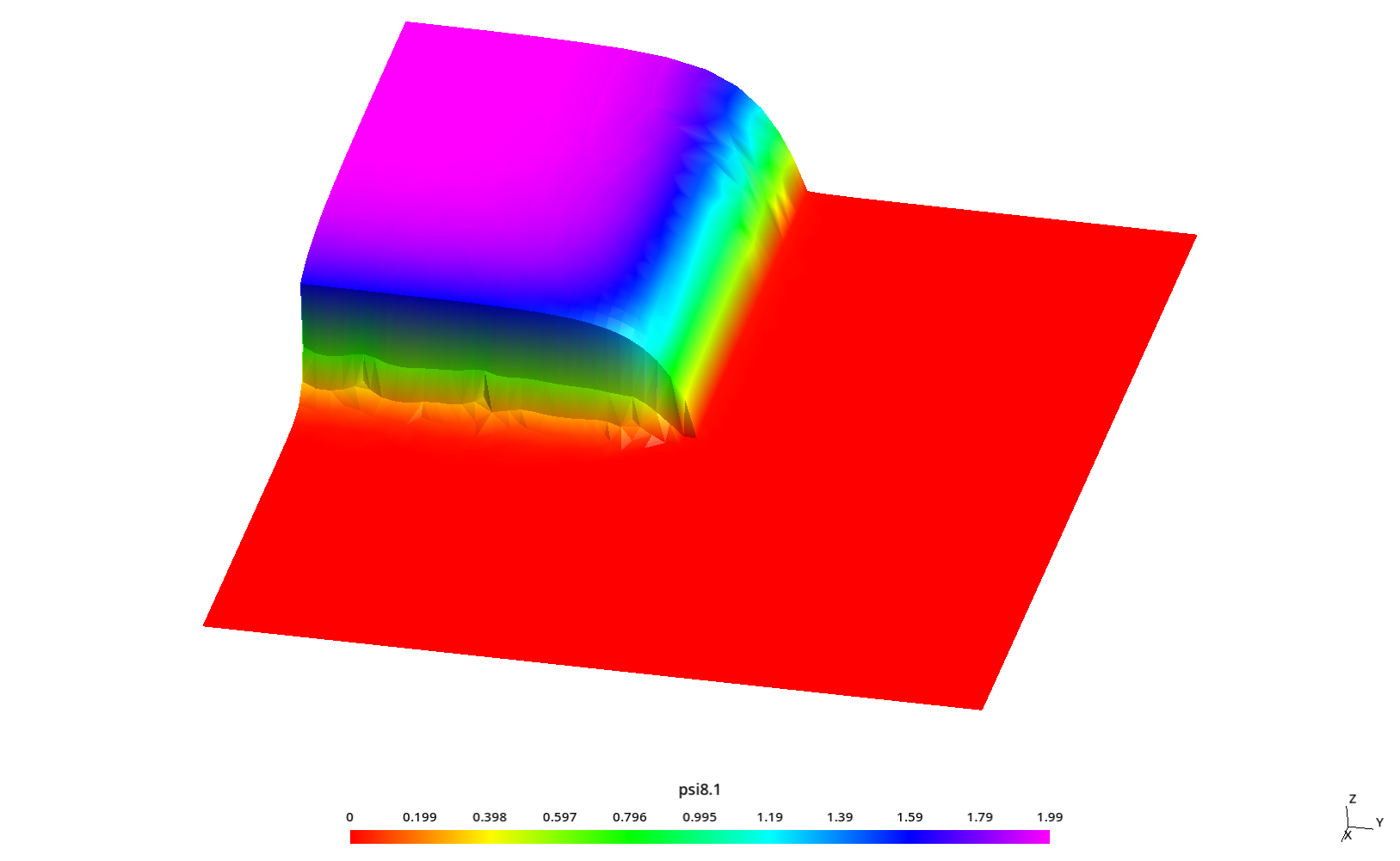\psi_{8,1} for S_4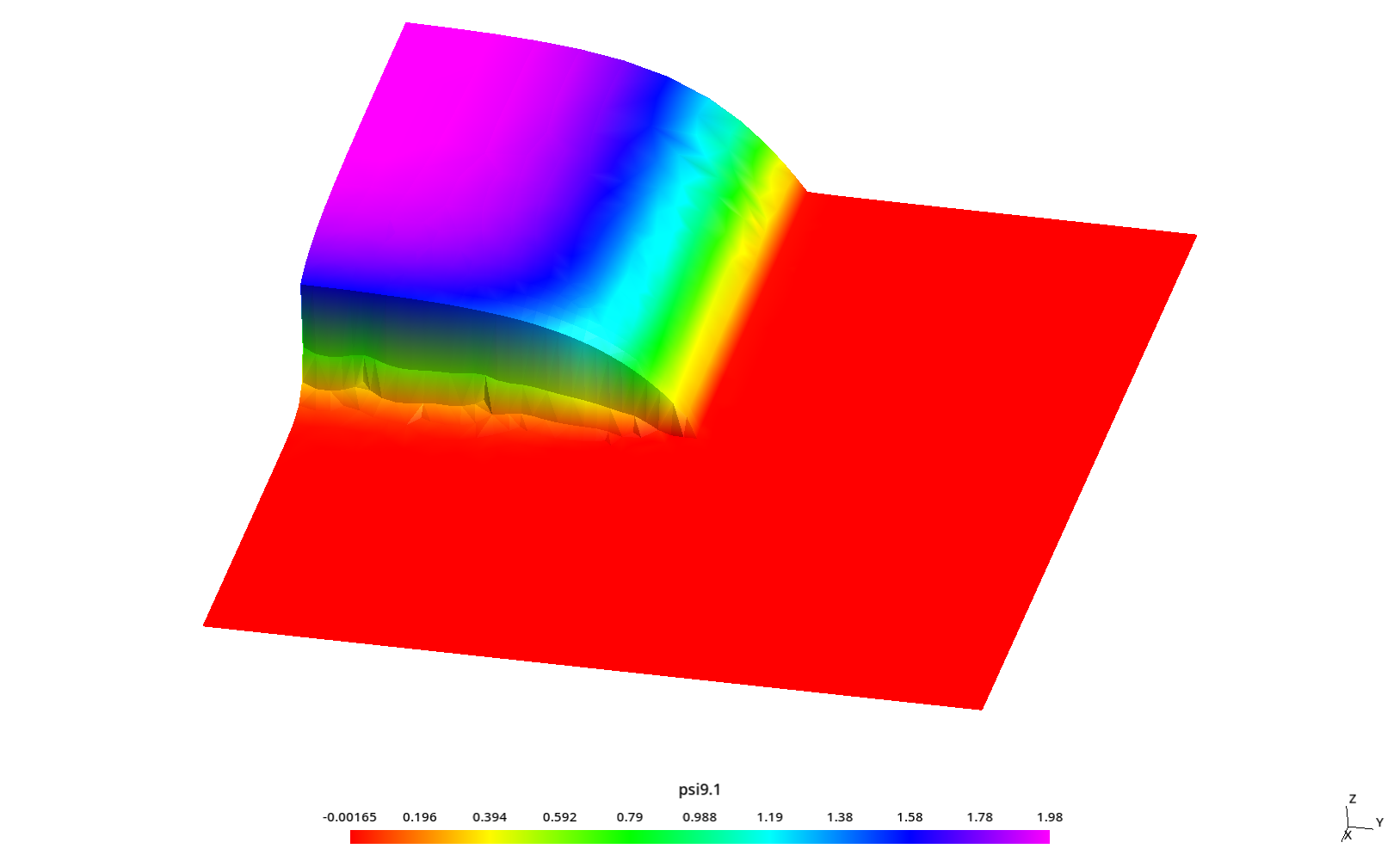\psi_{9,1} for S_4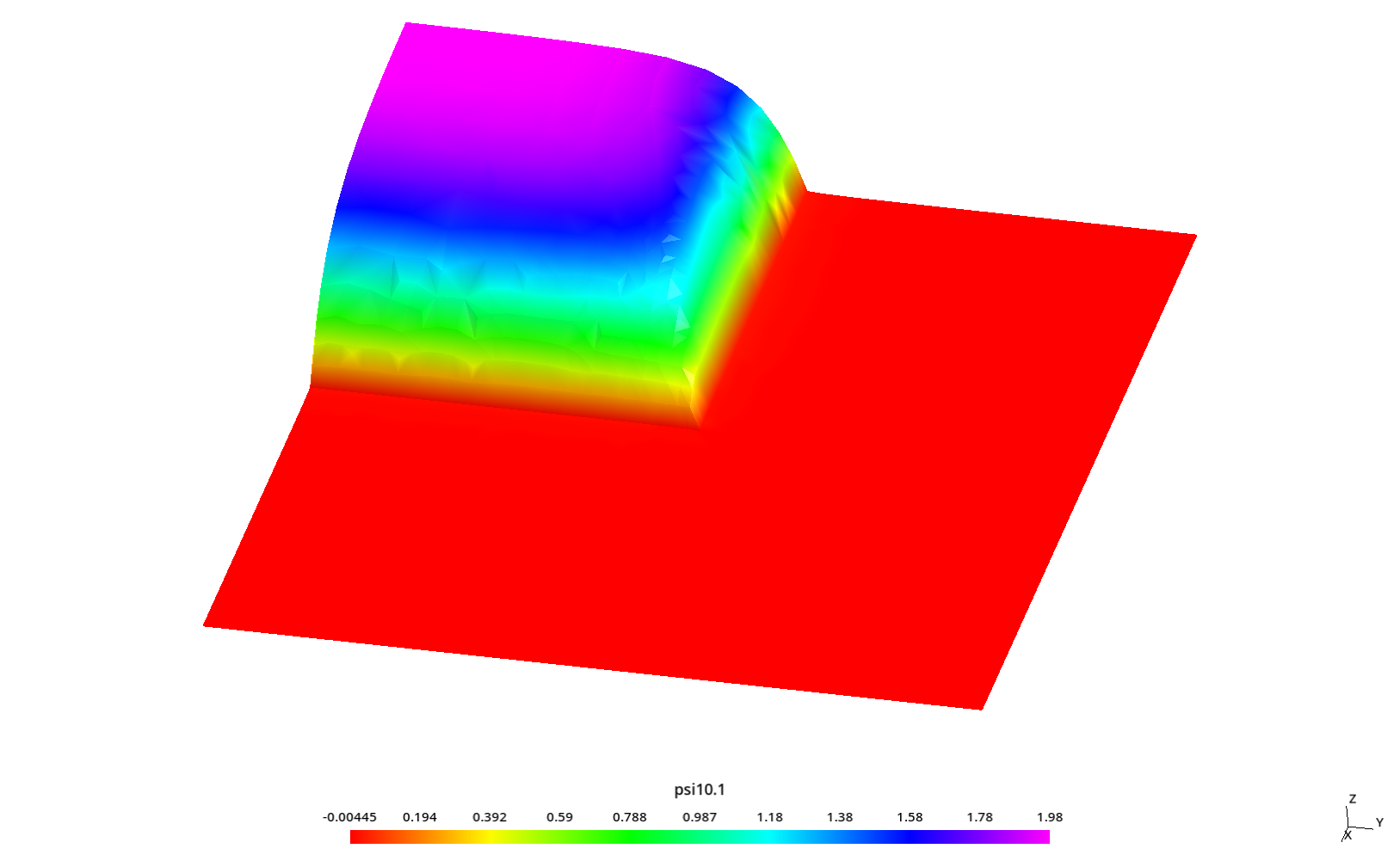\psi_{10,1} for S_4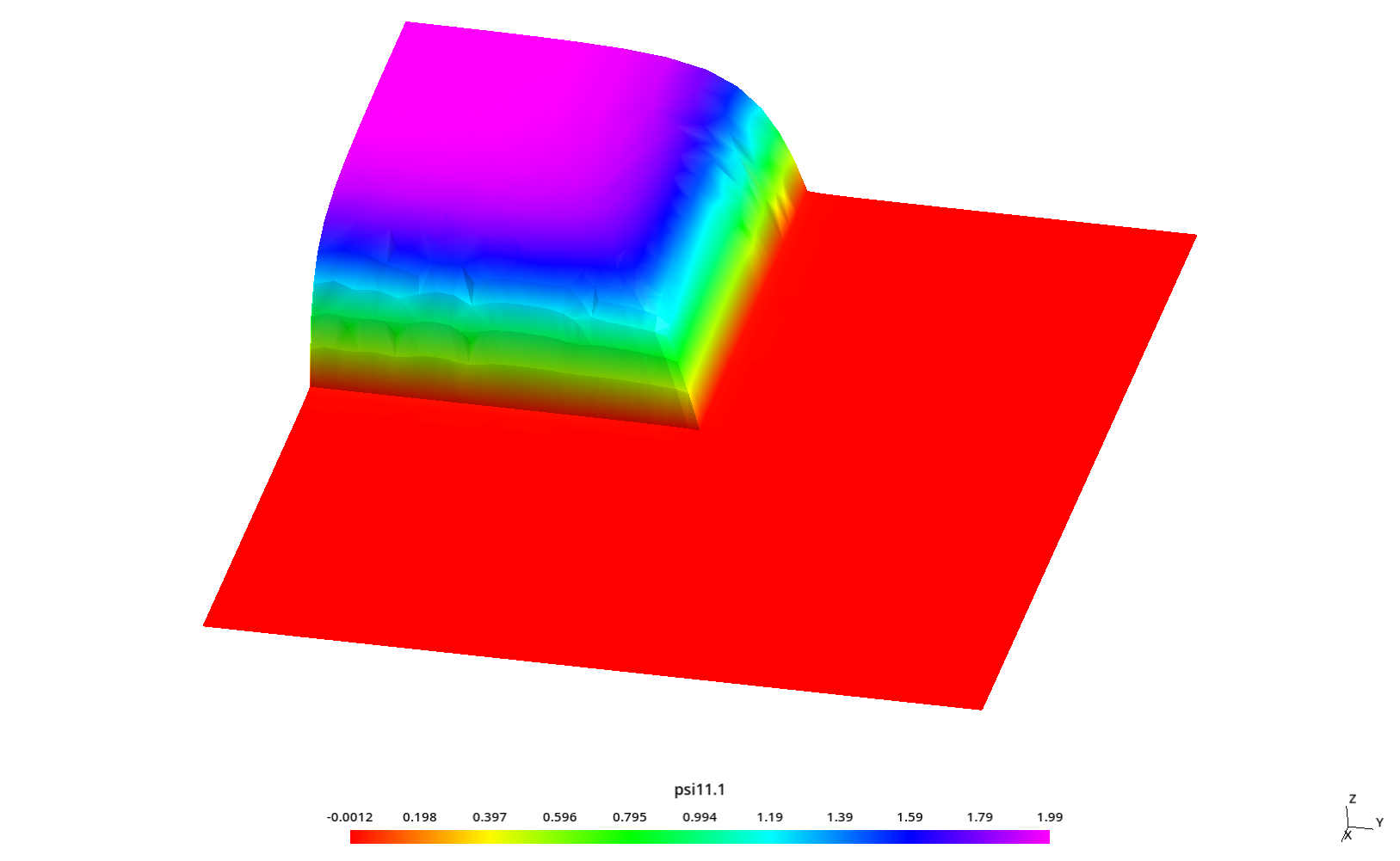\psi_{11,1} for S_4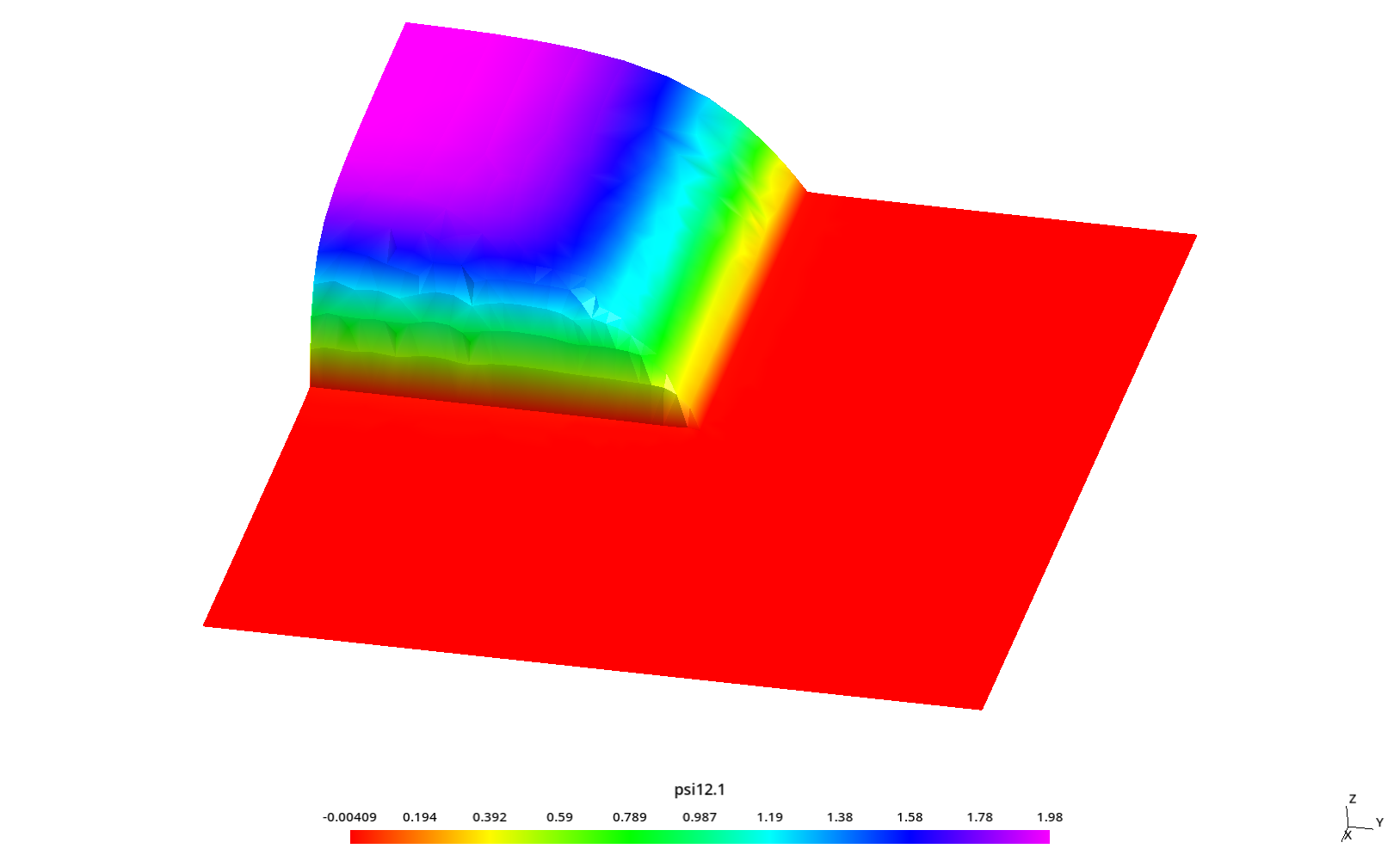\psi_{12,1} for S_4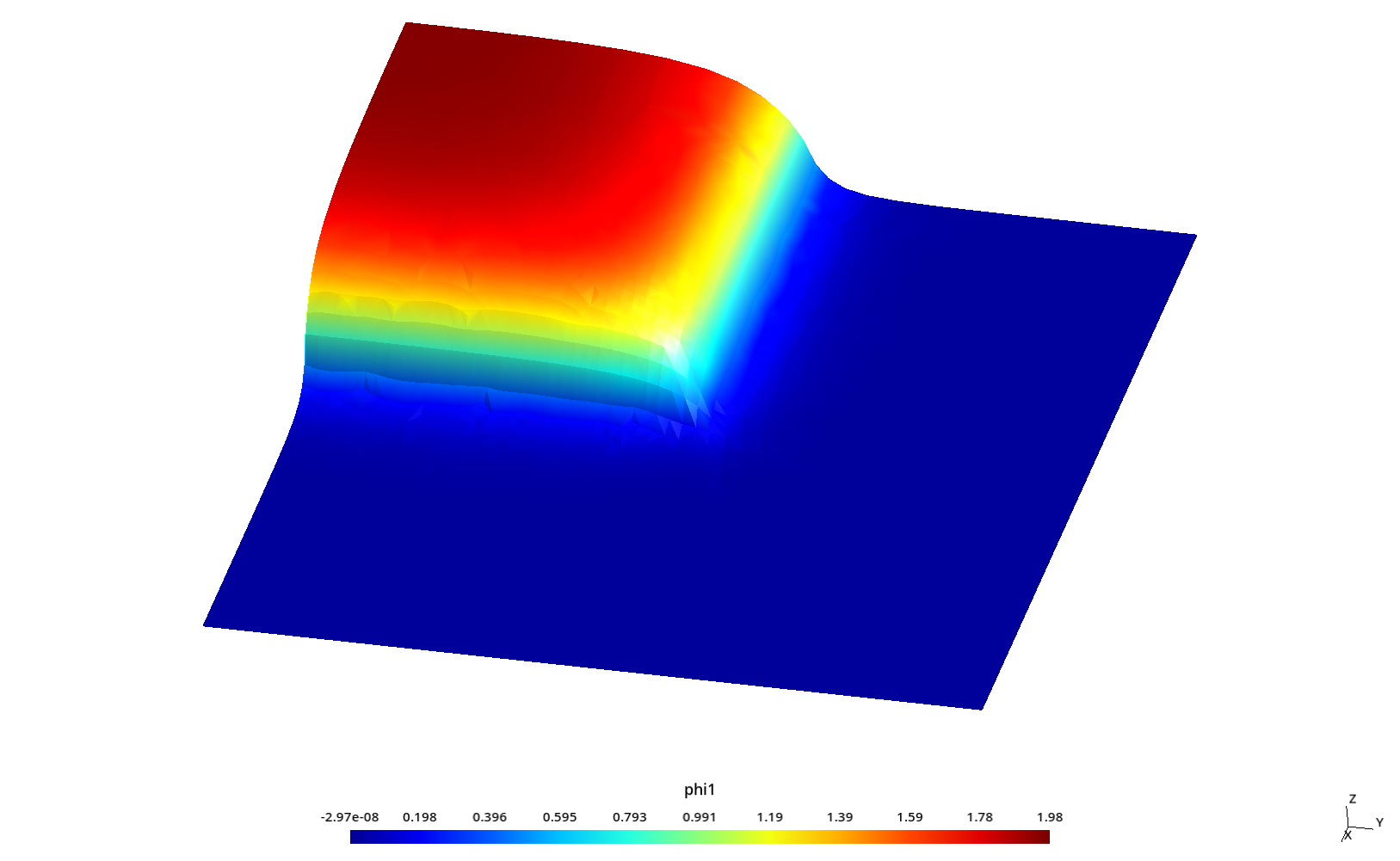\phi_{1} for S_4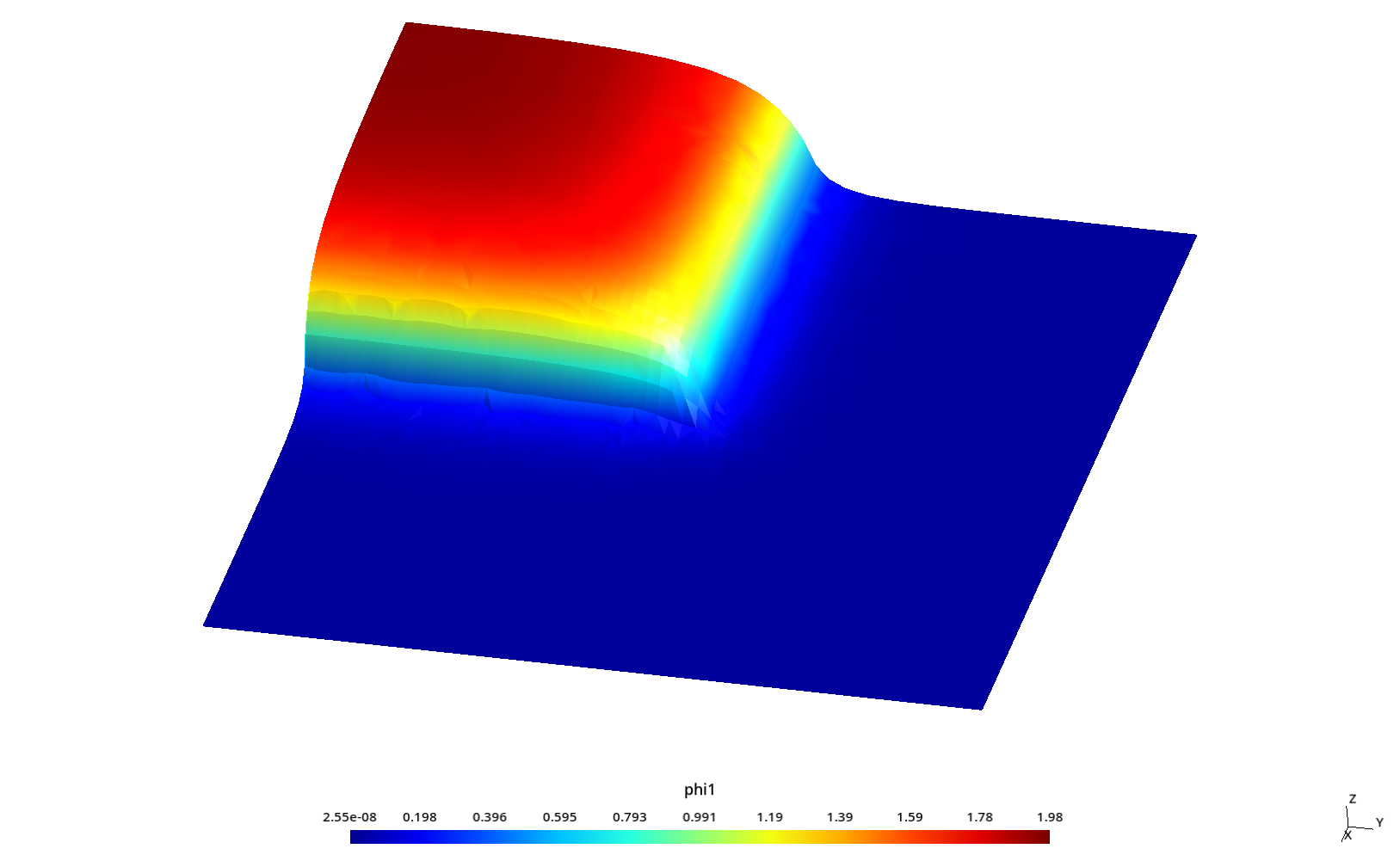\phi_{1} for S_6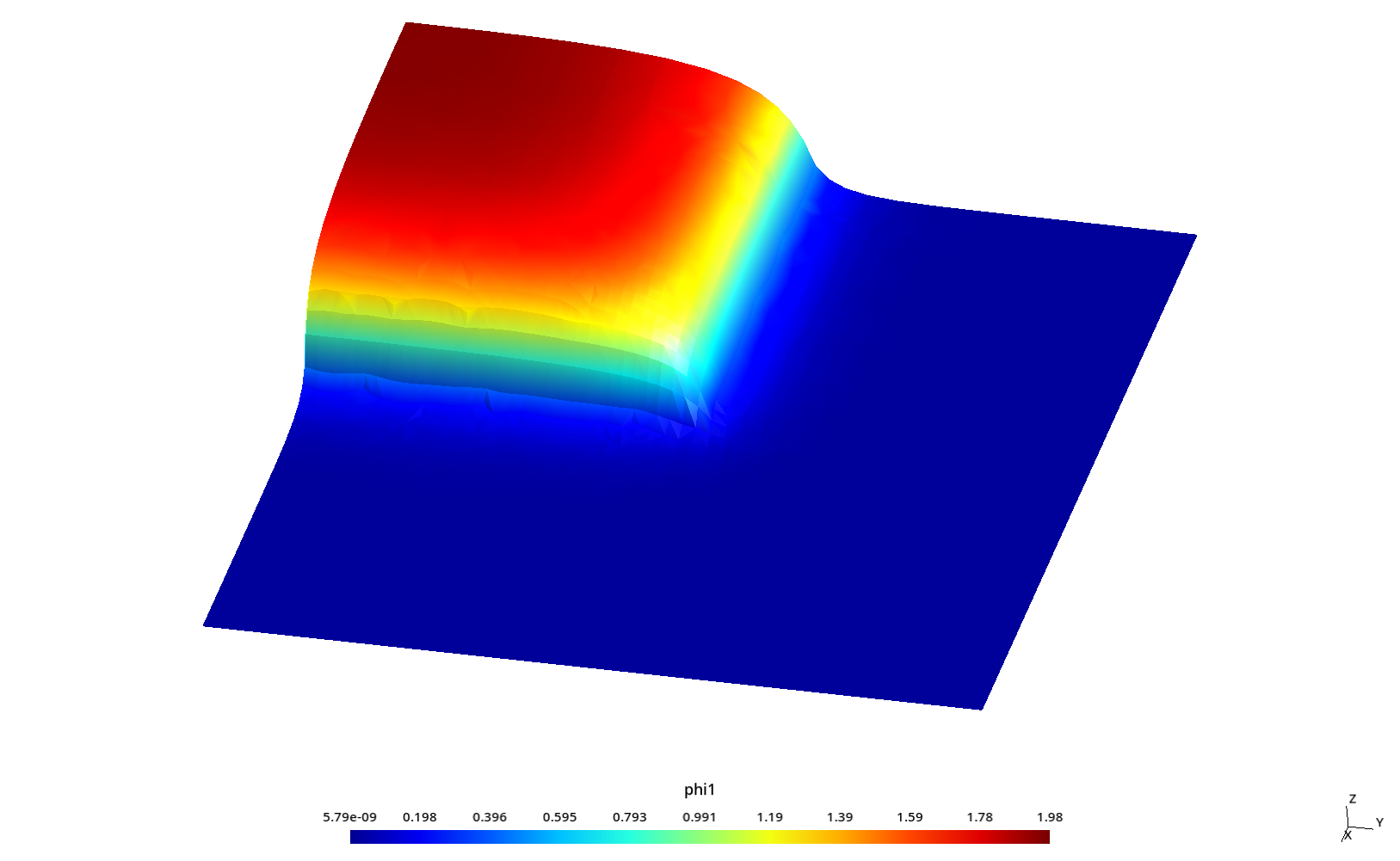\phi_{1} for S_8 ## 2.3 Flux profiles with ray effect This section analyzes flux profiles along the y axis at three different values of x as in section 6.4.1 of HyeongKae Park’s Master’s thesis, namely 1. x=5.84375 2. x=7.84375 3. x=9.84375 Some kind of “ray effect” is expected since the flux is not as large as in the core source section and the discrete numbers of neutron directions might induce numerical artifacts when evaluating the total scalar neutron flux. To better understand these profiles, the original square is rotated a certaing angle \theta \leq 45º around the z direction (coming out of the screen) keeping the S_N directions fixed. Since we cannot use mirror boundary conditions for an arbitrary \theta, we use the full geometry instead of only one quarter like in the two preceding sections. Therefore, we perform a parametric sweep over 1. the angle \theta of rotation of the original square in the x-y plane 2. a mesh scale factor c 3. N=4,6,8,10,12 #!/bin/bash thetas="0 15 30 45" cs="4 3 2 1.5 1" sns="4 6 8 10 12" for theta in${thetas}; do
echo "angle = ${theta};" > azmy-angle-${theta}.geo
for c in ${cs}; do gmsh -v 0 -2 azmy-angle-${theta}.geo azmy-full.geo -clscale ${c} -o azmy-full-${theta}.msh
for sn in ${sns}; do if [ ! -e azmy-full-${theta}-${sn}-${c}.dat ]; then
echo ${theta}${c} ${sn} feenox azmy-full.fee${theta} ${sn}${c} --progress
fi
done
done
done
DEFAULT_ARGUMENT_VALUE 1 0
DEFAULT_ARGUMENT_VALUE 2 4
DEFAULT_ARGUMENT_VALUE 3 0
PROBLEM neutron_sn DIM 2 GROUPS 1 SN $2 READ_MESH$0-$1.msh MATERIAL src S1=1 Sigma_t1=1 Sigma_s1.1=0.5 MATERIAL abs S1=0 Sigma_t1=2 Sigma_s1.1=0.1 BC vacuum vacuum sn_alpha = 0.5 SOLVE_PROBLEM theta =$1*pi/180
x'(d,x) = d*cos(theta) - x*sin(theta)
y'(d,x) = d*sin(theta) + x*cos(theta)

profile5(x) = phi1(x'(5.84375,x), y'(5.84375,x))
profile7(x) = phi1(x'(7.84375,x), y'(7.84375,x))
profile9(x) = phi1(x'(8.84375,x), y'(9.84375,x))

PRINT_FUNCTION profile5 profile7 profile9 MIN -10 MAX 10 NSTEPS 1000 FILE $0-$1-$2-$3.dat

# WRITE_RESULTS FORMAT vtk
PRINTF "%g unknowns for S${2} scale factor =${3}, memory needed = %.1f Gb\n" total_dofs memory()
# FILE res MODE "a" PATH azmy-resources.dat
# PRINT total_dofs wall_time() memory() $1$2 $3 FILE res $ ./azmy-full.sh
[...]
$pyxplot azmy-full.ppl$


There are lots (a lot) of results. Let’s show here a dozen to illustrate the ray effect.

Let’s start with \theta=0 (i.e. the original geometry) for N=4, N=8 and N=12 to see how the profiles “improve”: